# ISEE Lower Level Math : How to find the perimeter of a rectangle

## Example Questions

← Previous 1 3 4 5 6

### Example Question #1 : How To Find The Perimeter Of A Rectangle

Dennis is building a fence around his field to keep his cattle from getting off the property. If Dennis’ field ismiles long andmiles wide, how much fence will Dennis need to surround all of his property?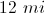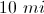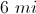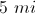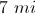Explanation:

In order to determine how much fence Dennis will need, we must find the perimeter of his property, which can be found using the formula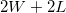. When we plug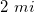in the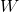and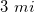in for, we find that Dennis needs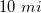of fence to surround his property.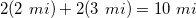### Example Question #2 : How To Find The Perimeter Of A Rectangle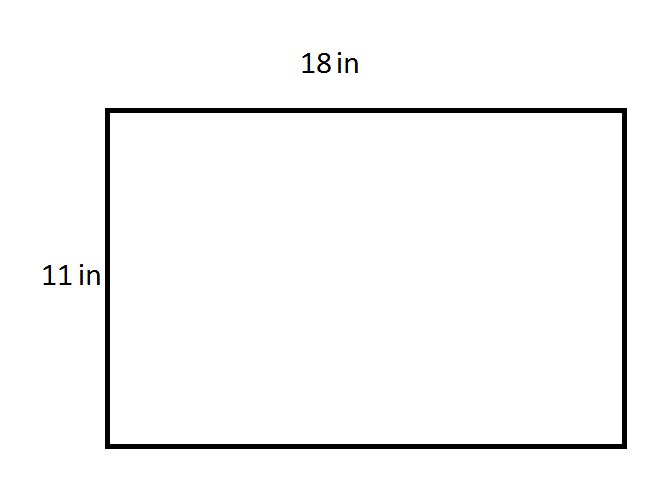Give the perimeter of the rectangle in the above diagram.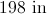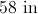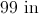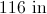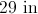Explanation:

Add the length and the height, then multiply the sum by two: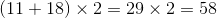The rectangle has a perimeter of 58 inches.

### Example Question #3 : How To Find The Perimeter Of A Rectangle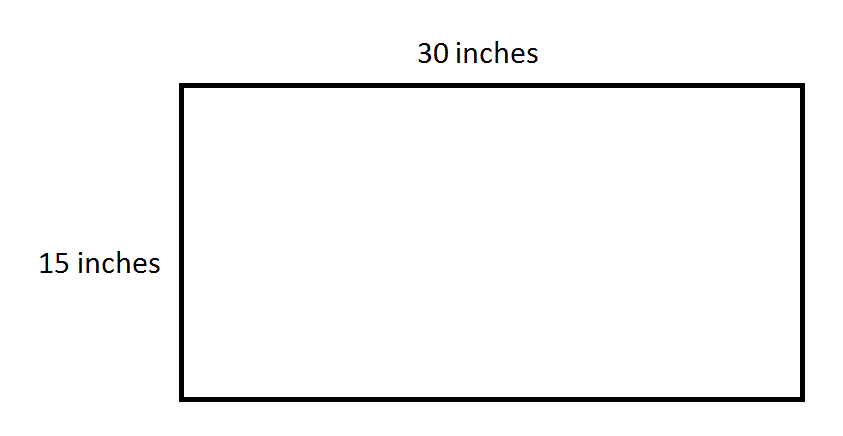Give the perimeter of the above rectangle in feet.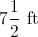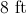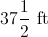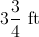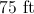Explanation:

Use the following formula, substituting: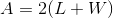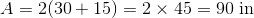Now, divide this by 12 to convert inches to feet.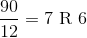6 inches make half of a foot, so this means the perimeter is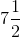feet.

### Example Question #4 : How To Find The Perimeter Of A Rectangle

What is the perimeter of a rectangle that has a length ofinches and a width ofinches?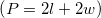Explanation:

The perimeter of a shape is the sum of all its sides. To find the perimeter of a rectangle, one can use the formula listed above,, since a rectangle has opposite sides of equal length.

The length of the rectangle is: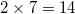The width of the rectangle is: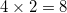.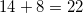Therefore, the perimeter is.

### Example Question #5 : How To Find The Perimeter Of A Rectangle

What is the perimeter of a rectangle with length equal to 5 and width equal to 3?

8

30

16

15

16

Explanation: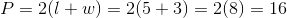### Example Question #6 : How To Find The Perimeter Of A Rectangle

What is the perimeter of a rectangle with a width ofand a length of?Explanation:

The perimeter of a rectangle is equal to the sum of all its sides. The formula for finding the perimeter of a rectangle is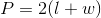The length of the rectangle is eight, and the width is five;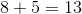.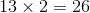Therefore, the perimeter of the rectangle is.

### Example Question #7 : How To Find The Perimeter Of A Rectangle

Mr. Barker is building a rectangular fence. His yard has an area offeet, and the one side of the fence he's already built isfeet long.

What will the perimeter of his fence be when he is finished building it?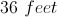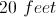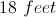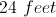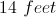Explanation:

The perimeter is calculated by adding up all the sides of the rectangle—in this case,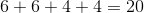So the perimeter isfeet.

### Example Question #8 : How To Find The Perimeter Of A Rectangle

A rectangle has sides 10 cm and 4 cm. What is its perimeter?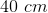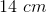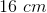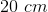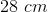Explanation:

A rectangle has two sides of congruent, or equal, sides. Therefore, there are two 10 cm sides and two 4 cm sides. Perimeters is the sum of all the sides, so you must add up the four sides. The answer is 28 cm.

### Example Question #9 : How To Find The Perimeter Of A Rectangle

If a rectangle has a width of 6.5 inches and a length of 9 inches, what is its perimeter?Explanation:

The perimeter of a rectangle is found by adding together all four sides. Two sides will be equal to the length, and two sides will be equal to the width.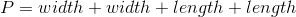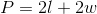Since the width is 6.5 inches and the length is 9 inches, the perimeter would be: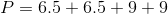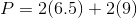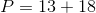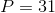### Example Question #10 : How To Find The Perimeter Of A Rectangle

If Margaret is buying a tablecloth for a table that is 4 feet by 2 feet, what should be the area of the tablecloth?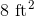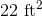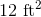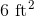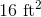Explanation:

We will need to find the area of the rectangle by multiplying the length by the width: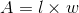Use the given dimensions: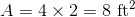← Previous 1 3 4 5 6